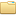## MIT OCW 6.006 - Introduction to Algorithms, Fall 2011 Demaine, Erik and Srinivas DevadasMIT6.006F11 (48 files)
Type: Course
Tags: mit, ocw

Bibtex:
```@article{,
title= {MIT OCW 6.006 - Introduction to Algorithms, Fall 2011},
journal= {MIT OpenCourseWare: Massachusetts Institute of Technology},
author= {Demaine, Erik and Srinivas Devadas},
year= {2011},
url= {http://ocw.mit.edu/courses/electrical-engineering-and-computer-science/6-006-introduction-to-algorithms-fall-2011},
abstract= {This course provides an introduction to mathematical modeling of computational problems. It covers the common algorithms, algorithmic paradigms, and data structures used to solve these problems. The course emphasizes the relationship between algorithms and programming, and introduces basic performance measures and analysis techniques for these problems.},